# Triangles

A triangle is composed of three line segments. The line segments intersect in their endpoints. To name a triangle we often use its vertices (the name of the endpoints). The triangle below is named ABC.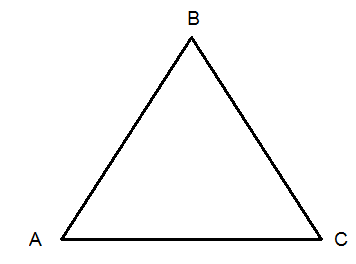A triangle has three angles. The sum of the measures of the angles is always 180° in a triangle.

We have different types of triangles. A triangle is classified by its angles and by the number of congruent sides.

A triangle that has three acute angels is called an acute triangle.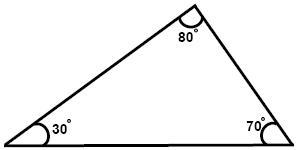A triangle that has one right angle is called a right triangle.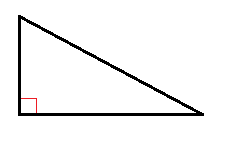A triangle that has one obtuse angle is called an obtuse triangle.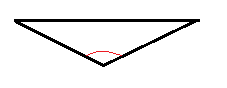When a triangle has three congruent sides, we call the triangle an equilateral triangle. We mark the congruent sides by a slash mark. The angles in an equilateral triangle are always 60°.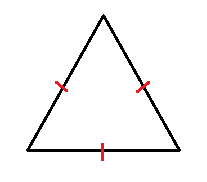When a triangle has two congruent sides it is called an isosceles triangle. The angles opposite to the two sides of the same length are congruent.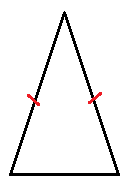A triangle without any congruent sides or angles is called a scalene triangle.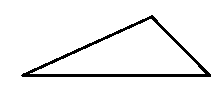When two triangles are congruent it means that they have the same size and shape. This means that they have the same angles. The red slash marks show us which sides and angles that are congruent Congruency is shown by this symbol

$\cong$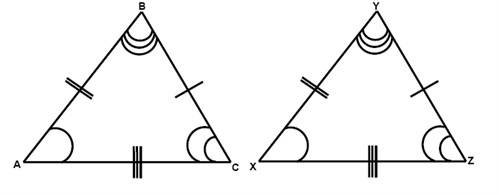$\begin{matrix} A\cong X & & AB\cong XY\\ B\cong Y & & BC\cong YZ\\ C\cong Z & & AC\cong XZ \end{matrix}$

Triangles that have congruent angles but not the same size are called similar. Similar triangles have sides that are proportional. Similarity is shown by this symbol

$\sim$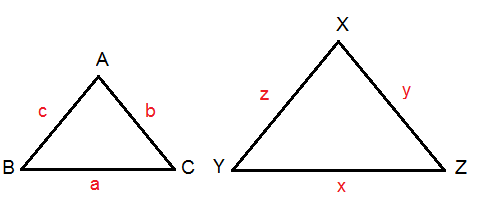$\bigtriangleup ABC\sim \bigtriangleup XYZ$

$A=X,\: \: B=Y,\: \: C=Z$

$\frac{a}{x}=\frac{b}{y}=\frac{c}{z}$

Example

Find x in the similar triangles.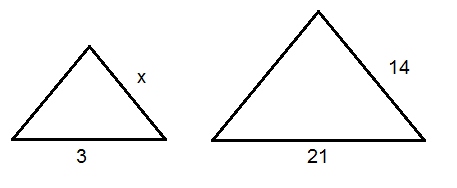We know that since the triangles are similar the sides are proportional which means that

$\frac{x}{14}=\frac{3}{21}\Rightarrow$

$x=\frac{14\cdot 3}{21}=\frac{42}{21}=2$

$x=2$

## Video lesson

Find out whether the triangles are right, isosceles, acute, scalene, obtuse or equilateral